### IMO Shortlist 2007 problem G2

Kvaliteta:
Avg: 0.0
Težina:
Avg: 6.0
Denote by$M$ midpoint of side$BC$ in an isosceles triangle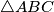$\triangle ABC$ with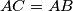$AC = AB$. Take a point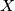$X$ on a smaller arc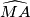$\widehat{MA}$ of circumcircle of triangle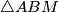$\triangle ABM$. Denote by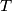$T$ point inside of angle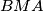$BMA$ such that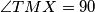$\angle TMX = 90$ and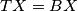$TX = BX$.

Prove that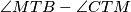$\angle MTB - \angle CTM$ does not depend on choice of$X$.